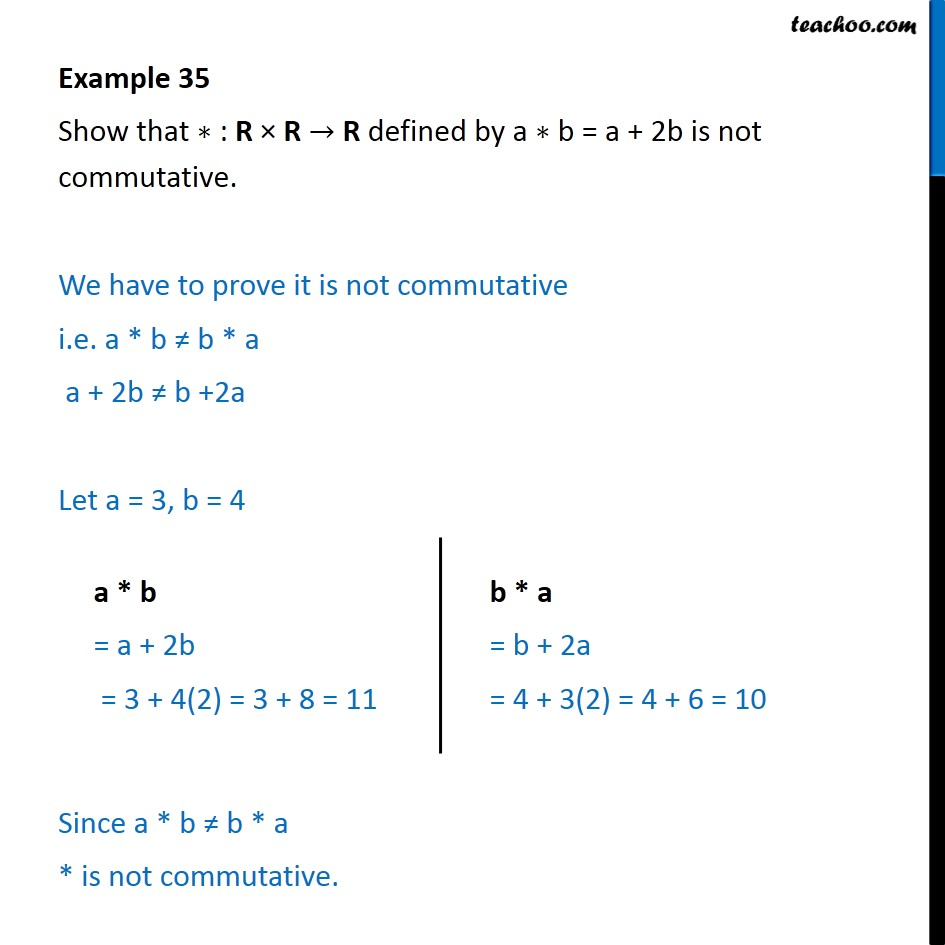Subscribe to our Youtube Channel - https://you.tube/teachoo

1. Chapter 1 Class 12 Relation and Functions
2. Serial order wise
3. Examples

Transcript

Example 35 Show that ∗ : R × R → R defined by a ∗ b = a + 2b is not commutative. We have to prove it is not commutative i.e. a * b ≠ b * a a + 2b ≠ b +2a Let a = 3, b = 4 Since a * b ≠ b * a * is not commutative.

Examples